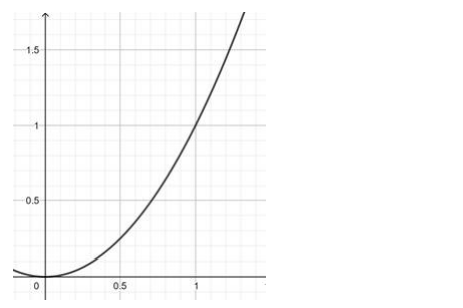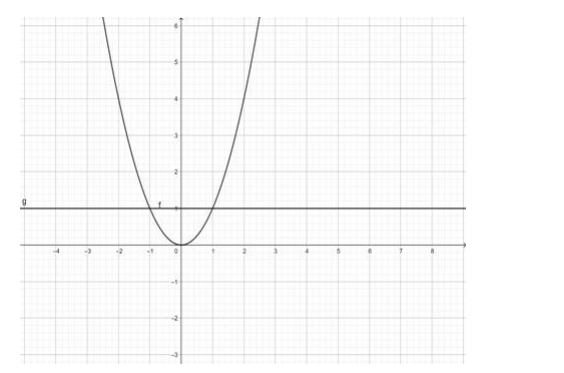# Show that the functionQuestion:

Show that the function

(i) $f: N \rightarrow N: f(x)=x^{2}$ is one - one into.

(ii) $f: Z \rightarrow Z: f(x)=x^{2}$ is many - one into

Solution:

(i) $f: N \rightarrow N: f(x)=x^{2}$ is one - one into.

$f(x)=x^{2}$

$\Rightarrow y=x^{2}$Since the function $f(x)$ is monotonically increasing from the domain $N \rightarrow N$

$\therefore f(x)$ is one -one

Range of $f(x)=(0, \infty) \neq N($ codomain $)$

$\therefore f(x)$ is into

$\therefore f: N \rightarrow N: f(x)=x^{2}$ is one - one into.

(ii) $f: Z \rightarrow Z: f(x)=x^{2}$ is many - one into

$f(x)=x^{2}$

$\Rightarrow y=x^{2}$

in this range the lines cut the curve in 2 equal valued points of $y$, therefore, the function $f(x)=x^{2}$ is many one.

Range of $f(x)=(0, \infty) \neq Z$ (codomain)

$\therefore f(x)$ is into